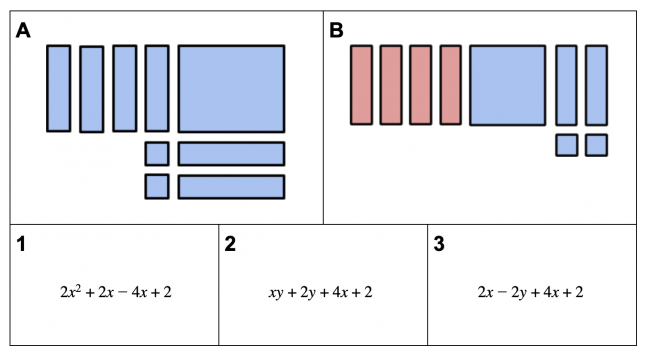## You are here

Preview:This task helps students connect different polynomial expressions with visuals representing polynomials.

Connecting Representations
Big Idea:
Grade 9: The principles and processes underlying operations with numbers apply equally to algebraic situations and can be described and analyzed.
Content:
operations with polynomials, of degree less than or equal to 2
Curricular Competency:
Reasoning and analyzing: Use reasoning to explore and make connections
Understanding and solving: Develop, demonstrate, and apply mathematical understanding through play, inquiry, and problem solving
Understanding and solving: Visualize to explore mathematical concepts
Communicating and representing: Communicate mathematical thinking in many ways